# AP Board 9th Class Physical Science Solutions Chapter 5 What is inside the Atom?

AP State Syllabus AP Board 9th Class Physical Science Solutions Chapter 5 What is inside the Atom? Textbook Questions and Answers.

## AP State Syllabus 9th Class Physical Science Solutions 5th Lesson What is inside the Atom?

### 9th Class Physical Science 5th Lesson What is inside the Atom? Textbook Questions and Answers

Question 1.
What are the three subatomic particles? (AS 1)
The three sub-atomic particles are electrons, protons, and neutrons.

Question 2.
Compare the subatomic particles electron, proton, and neutron. (AS 1)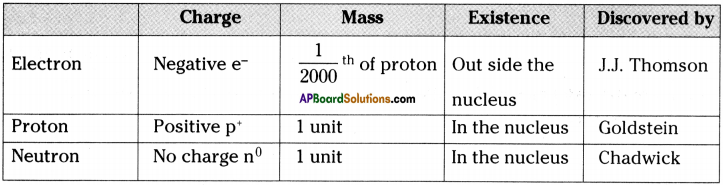Question 3.
What are the limitations of J.J. Thomson’s model of the atom? (AS 1)
The main limitation of J.J. Thomson’s model of atom was that he is unable to explain, how the positively charged particles are shielded from negatively charged particles without getting neutralized.Question 4.
What were the three major observations Rutherford made in the gold foil experiment? (AS 1)
The three major observations Rutherford made in the gold foil experiment were

1. Most of the space inside the atom is empty.
2. All the positive charge must be concentrated in a very small space within the atom called nucleus.
3. The size of the nucleus is very small as compared to the size of the atom.

Question 5.
Sketch Rutherford’s atomic model. Why is Rutherford’s model of the atom called the planetary model? (AS 5)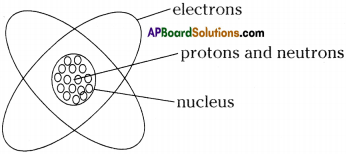Rutherford’s model is called planetary model because the motion of the electrons around the nucleus resembles the motion of the planets around the sun.

Question 6.
Put tick (✓) against correct choice and cross (✗) against wrong choice: (AS 1)
i) In Rutherford’s gold foil experiment, majority of alpha particles passed directly through the gold foil. This observation leads to which conclusions?
a) The positively charged region of the atom is very small. (✗)
b) The atom must consist of empty space. (✓)
c) The alpha particles makes a direct hit on the positively charged region of the atom. (✗)
d) The positively charged region of the atom is very dense. (✗)

ii) In Rutherford’s gold foil experiment, occasionally the alpha particles veered from a straight line path. This observation leads to which conclusion?
a) The positively charged region of the atom is very small. (✗)
b) The majority of the space in the atom is empty. (✗)
c) The alpha particle makes a direct hit on the positively charged region of the atom. (✗)
d) The positively charged region of the atom is very dense. (✓)Question 7.
Which one of the following is the correct electronic configuration of sodium? (AS 1)
a) 2, 8
b) 8, 2, 1
c) 2, 1, 8
d) 2, 8, 1
d) 2, 8, 1

Question 8.
Give the main postulates of Bohr’s model of an atom. (AS 1)
The main postulates of Bohr’s model of atom are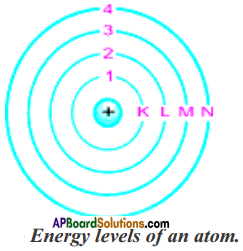1. Only certain special, discrete orbits of electrons are allowed inside the atom. These orbits or shells are called energy levels.
2. While revolving in these discrete orbits the electrons do not radiate energy and this helps that the electrons do not crash into the nucleus.
3. These orbits or shells are represented by the letters K, L, M, N, ……… or the numbers n = 1, 2, 3, …………Question 9.
Compare all the proposed models of an atom given in this chapter. (AS 1)
In this chapter, four atomic models were discussed. The main postulates of those models are

1. Dalton’s proposal :
a) Atoms are indivisible.
b) Atoms of an element are all identical to each other and different from the atoms of other elements.

2. Thomson’s proposal:
a) An atom is considered to be a sphere of uniform positive charge and electrons are embedded into it.
b) The total mass of the atom is considered to be uniformly distributed throughout the atom.

3. Rutherford’s proposal:
a) All the positively charged material in an atom formed a small dense centre, called the nucleus of the atom.
b) Negatively charged electrons revolve around the nucleus in well defined orbits.
c) Size of the nucleus is very small as compared to the size of the atom.

4. Neils Bohr’s proposal :
a) Electrons are revolving around the nucleus in special, discrete orbits called en¬ergy levels or shells.
b) While revolving in these discrete orbits the electron do not radiate energy and this helps that the electrons do not crash into the nucleus.
c) These orbits or shells are represented by the letters K, L, M, N,…. or the numbers n = 1, 2, 3,Question 1o.
Define valency by taking examples of nitrogen and boron. (AS 1)
Valency: The number of electrons present in outer most orbit of an atom is called its valency. Valency of Nitrogen :
a) Atomic number of nitrogen is 7.
b) The distribution of electrons is 2, 5.
c) The outer most orbit has 5 electrons.
d) Hence its valency should be 5. But it is easier to nitrogen to gain 3 electrons than to loose 5 electrons for becoming octet.
e) Hence the valency of nitrogen is ‘3’.

Valency of Boron :
a) Atomic number of boron is 5.
b) The distribution of electrons is 2, 3.
c) The outer most orbit has 3 electrons.
d) Hence the valency of boron is 3.

Question 11.
State the valencies of the following elements : magnesium and sodium. (AS 1)
Magnesium :
a) Atomic number of magnesium is 12.
b) Distribution of electrons is 2, 8, 2.
c) Hence the valency is 2.

Sodium :
a) Atomic number of sodium is 11.
b) Distribution of electrons is 2, 8, 1.
c) Hence the valency is 1.

Question 12.
If Z = 5, what would be the valency of the element? (AS 2)
1) If Z = 5, the distribution of electrons is 2, 3.
2) Hence the valency is ‘3’.

Question 13.
Write the atomic number and the symbol of an element which has mass number 32 and the number of neutrons 16 in the nucleus. (AS 1)
Mass number (A) = 32 ; Number of neutrons (N) = 16
Number of protons (Z) = A- N = 32-16 = 16
∴ Atomic number =16
The element is sulphur.
The symbol of sulphur is S’.

Question 14.
Cl- has completely filled K and L shells. Explain. (AS 1)
Atomic number of Cl is 17, but Cl has one more electron when compared with Cl atom. Distribution of electrons in Cl is

 K L M 2 8 8

K shell can accommodate 2 electrons and L shell cab accommodate 8 electrons accord¬ing to the formula 2n2.
Hence the K and L shells are completely filled.

Question 15.
What is the main difference among the isotopes of the same element? (AS 1)
The main difference between isotopes of the same element is
a) The number of neutrons is different.
b) Their physical properties are different but the chemical properties are similar.

Question 16.
For the following statements, write T for True and F for False. (AS 1)
a) J.J. Thomson proposed that the nucleus of an atom contains only nucleons.
b) A neutron is formed by an electron and a proton combining together. Therefore, it is neutral.
c) The mass of an electron is 1836 times that of proton.
a) False
b) False
c) True

Question 17.
Fill in the missing information in the following table. (AS 4)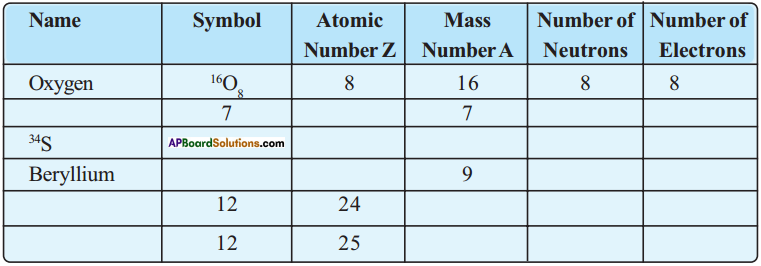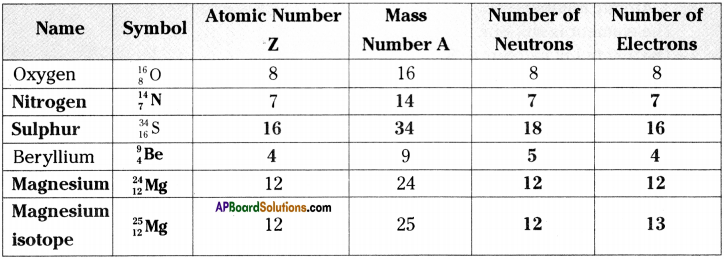Question 18.
How do you appreciate the efforts made by scientists to explain the structure of atom by developing various atomic models? (AS 6)

• Structure of atom, till today it is mysterious and challenging the scientists.
• We have to appreciate the scientists right from Lavoisier, who proposed law of conservation of mass, Proust who proposed law of constant proportions, John Dalton for his first model of atom, Rutherford for giving planetary model of atom and Neils Bohr for his model of atom.
• Till today scientists are trying to know the existence of more and sub-atomic particles besides electrons, protons and neutrons.
• Hence the efforts of scientists are highly appreciable, for making our lives comfortable and leaving many challenges before us to unveil or discover them.

Question 19.
Geeta got a doubt, “Why does atomic nucleus contain proton and neutrons? Why can’t electrons and neutrons be in it”. Can you help to clarify her doubt? Explain. (AS 1)
Nucleus contains protons and neutrons inside it but not electrons and neutrons. If it would have happen, then

1. the alpha particles in the Rutherford’s alpha particle scattering experiment would have not been deflected or scattered.
2. the idea of nucleus would have not been evolved because the mass of electron is negligible, it is most unstable.Question 20.
Collect information about various experiments conducted and theories proposed by scientists starting from John Dalton to Neils Bohr. Prepare a story with a title “History of atom”. (AS 4)
History of atom
John Dalton proposed atomic theory based on law of conservation of mass and law of constant proportion as :

1. Atoms were indivisible.
2. Atoms of an element are all identical to each other and different from the atoms of other elements.

Later on various experiments conducted by Thomson, Goldstein, etc. proved that atom is divisible and consists sub-atomic particles like electrons, protons and neutrons. Based on this J.J. Thomson proposed a model of atom in 1898.

According to Thomson,

1. An atom is considered to be a sphere of uniform positive charge and electrons are embedded into it.
2. The total mass of the atom is considered to be uniformly distributed throughout the atom.
3. The negative and the positive charges are supposed to be balance out and the atom as a whole is electrically neutral.

This model is also called as plum pudding model or watermelon model.

Thomson’s student Ernest Rutherford conducted alpha particle scattering experiment and got the results which were not in favour of Thomson’s model. Based on his experiment, Rutherford proposed a model of atom. According to him,

1. All the positively charged material in an atom formed a small dense centre, called the nucleus of the atom. The electrons were not a part of nucleus.
2. Negatively charged electrons revolve around the nucleus in well – defined orbits like planets revolve around the sun.
3. The size of nucleus is very small as compared to the size of the atom.

This model could not account for stability of atom, as revolving electron must lose energy and eventually crash into the nucleus, as a result matter would not exist in the form that we see it now.

In 1913, Neils Bohr proposed another model to overcome Rutherford’s defect. According to Bohr,

1. Only certain special, discrete orbits of electrons are allowed in side the atom. These orbits or shells are called energy levels.
2. While revolving in these discrete orbits the electrons do not radiate energy and this helps that the electrons do not crash into the nucleus.
3. These orbits or shells are represented by K, L, M, N ………… or the numbers 1, 2, 3,

This model could not predict the spectra of atoms.

Hence this journey continues

### 9th Class Physical Science 5th Lesson What is inside the Atom? InText Questions and Answers

9th Class Physical Science Textbook Page No. 75

Question 1.
Why are the atoms of different elements different?
Nature and properties of elements depends on the arrangement of atoms. We know that different elements behave differently. This is due to the difference in their atoms.

Question 2.
Is there anything inside atom that make them to be same or different?
The arrangement of sub atomic particles inside the atom is responsible to make them to be same or different.Question 3.
Are atoms indivisible?
No, atom is divisible. There are many sub-atomic particles inside the atom according to the recent experiments.

9th Class Physical Science Textbook Page No. 77

Question 4.
If an atom consists of sub-atomic particles like protons, neutrons and electrons, how are they arranged in the atom ?
The arrangement of sub-atomic particles like protons, neutrons and electrons has been explained by many scientists like Rutherford, Neils Bohr, etc. According to them, atom consists a central mass called nucleus. Nucleus consists protons and neutrons. Electrons revolve around the nucleus in fixed shells.

9th Class Physical Science Textbook Page No. 80

Question 5.
Why is atom stable?
In an atom, the number of protons in the nucleus is equal to the number of electrons out side the nucleus. Hence the positive and negative charges in an atom are equal. So, atom is electrically neutral. So, atom is stable. But the stability of atom was explained by Neils Bohr in a different way.

Question 6.
Can you suggest any other arrangement of subatomic particles in the atom which prevents the revolving electron to fall into the nucleus?
Electrons have to revolve around the nucleus in definite orbits such that the centripetal and centrifugal forces acting on the electron must be equal in magnitude and opposite direction. Then the revolving electron do not fall into the nucleus.

9th Class Physical Science Textbook Page No. 82

Question 7.
How many electrons can be accommodated in each shell of an atom?
The number of electrons that can be accommodated in each shell of an atom depends on the shell number. First shell (K) consists 2 electrons, second (L) shell consists 8 electrons, third (M) shell consists 18 electrons, fourth shell (N) consists 32 electrons, and so on.

Question 8.
Can a particular shell have just one electron?
No, shell has just one electron.Question 9.
What is the criteria to decide number of electrons in a shell?
The number of electrons in a shell can be decided by using a formula 2n². (Where n is shell number).

9th Class Physical Science Textbook Page No. 83

Question 10.
What is the valency of oxygen that you can calculate by the method discussed above?

• Oxygen has 8 electrons in its atom. The distribution of electrons is 2, 6.
• The outer most shell consists 6 electrons, this number is hear to 8.
• Hence the valency of oxygen is 8 – 6 = 2.

9th Class Physical Science Textbook Page No. 85

Question 11.
Should we consider the number of neutrons as a characteristic of an atom?
The mass of an atom which is a characteristic of an atom depends on the number of neutrons and protons that its nucleus contains. Hence the number of neutrons can be considered as a characteristic of an atom.

9th Class Physical Science Textbook Page No. 76

Question 12.
An atom is electrically neutral. But the electrons present in it are negatively charged particles. If only negative charges were present, the atom would not be neutral. Then, why are atoms considered to be neutral?

• This is the idea before Rutherford’s model.
• According to Rutherford’s model, number of protons inside the nucleus and number of electrons outside the nucleus are equal.
• Hence the net negative charge is equal to net positive charge. So, the atom is electrically neutral.

9th Class Physical Science Textbook Page No. 80

Question 13.
Compare Rutherford and Thomson’s models of the atom on the following basis :
1) Where is the positive charge placed?
2) How are the electrons placed?
3) Are they stationary inside the atom or moving?

1. According to Thomson, the positive charge is uniformly distributed throughout the atom. Whereas according to Rutherford, the positively charged protons are inside the nucleus.
2. According to Thomson, electrons are embedded in the positively charged atom, but according to Rutherford, electrons are revolving around the nucleus in welldefined orbits.
3. According to Thomson, electrons are stable inside the atom but according to Rutherford, electrons are moving inside the atom.

9th Class Physical Science Textbook Page No. 83

Question 14.
Phosphorus and sulphur show multiple valency. See table 2. Why do some elements show multiple valency? Discuss with your Mends and teachers.

• For sulphur, the number of electrons in outer most orbit is 6.
• Hence the valency should be (8 – 6 =) 2.
• But sulphur exists in so many forms.
• In the excited state, these 6 electrons also tend to participate in the bond formation.
• Hence sometimes it shows the valency 6. Ex : SO2, SO3, etc.
• Same situation happens for phosphorus. Ex . PCl3, PCl5, etc.

9th Class Physical Science 5th Lesson What is inside the Atom? Activities

Activity – 1

Question 1.
Sketch the structure of atom as you imagine.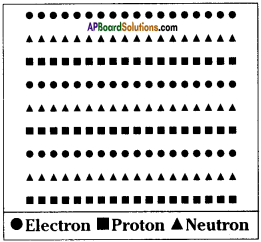We learnt about electron, proton and neutron.
a) Suppose you had to arrange them in an atom, how do you do it?